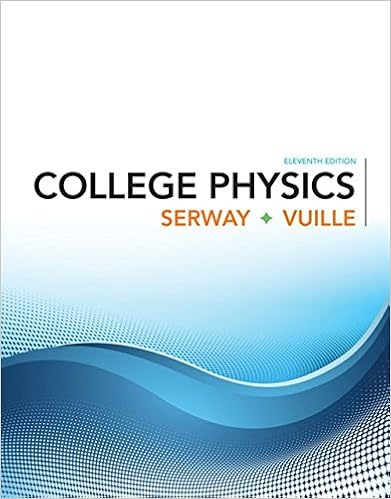# hw6sol-2017.pdf - Homework 6 Solution EE263 Stanford...

• Homework Help
• 15
• 100% (10) 10 out of 10 people found this document helpful

This preview shows page 1 - 4 out of 15 pages.

##### We have textbook solutions for you!
The document you are viewing contains questions related to this textbook.The document you are viewing contains questions related to this textbook.
Chapter 15 / Exercise 35
College Physics
Serway/VuilleExpert Verified
Homework 6 SolutionEE263 Stanford University, Fall 2017Due: Friday 11/17/17 11:59pm1. Harmonic oscillator.The system˙x=0ω-ω0xis called aharmonic oscillator.a) Find the eigenvalues, resolvent, and state transition matrix for the harmonic oscillator.Expressx(t)in terms ofx(0).b) Sketch the vector field of the harmonic oscillator.c) The state trajectories describe circular orbits,i.e.,kx(t)kis constant. Verify this factusing the solution from part (a).d) You may remember that circular motion (in a plane) is characterized by the velocityvector being orthogonal to the position vector. Verify that this holds for any trajectoryof the harmonic oscillator.Use only the differential equation; do not use the explicitsolution you found in part (a).Solution.
1
##### We have textbook solutions for you!
The document you are viewing contains questions related to this textbook.The document you are viewing contains questions related to this textbook.
Chapter 15 / Exercise 35
College Physics
Serway/VuilleExpert Verified
-10-8-6-4-20246810-10-8-6-4-20246810Figure 1: Vector field of harmonic oscillator2. Real modal form.We learned about the modal form of a system in class. Show that whensome of eigenvalues of the dynamics matrixAare complex, the system can be put inrealmodal form(Assuming the eigenvectors ofAare independent):S-1AS= diag (Λr, Mr+1, Mr+3, . . . , Mn-1)whereΛr= diag(λ1, . . . , λr)are the real eigenvalues, andMj=σjωj-ωjσj,λj=σj+j,j=r+ 1, r+ 3, . . . , n-1whereλjare the complex eigenvalues (one from each conjugate pair). Clearly explain whatthe matrixSis.Generate a matrixAinR10×10usingA=randn(10). (The entries ofAwill be drawn froma unit normal distribution.) Find the eigenvalues ofA. If by chance they are all real, generatea new instance ofA. Find the real modal form ofA,i.e., a matrixSsuch thatS-1AShasthe real modal form. Your solution should include the source code that you use to findS, andsome code that checks the results (i.e., computesS-1ASto verify it has the required form).Solution.AssumingAis diagonalizable, it can be written asA=TΛT-1, HereΛ = diag(λ1, . . . , λr, λr+1, . . . , λn),whereλ1, . . . , λrare the real eigenvalues ofAandλr+1, . . . , λnare the complex eigenvalues ofAand come in complex conjugate pairs. Lettibe theith column ofT. TakeSto beS= [t1· · ·tr<(tr+1)=(tr+1)· · · <(tn-1)=(tn-1)].
2
Let us now prove why constructingSin this way will give us the desired result.Letv=<(v) +i=(v)be a complex eigenvector ofAassociated with the eigenvalueλ=σ+.We must haveAv=λv,i.e.,A(<(v) +i=(v)) = (σ+)(<(v) +i=(v)).This implies thatA<(v) =σ<(v)-ω=(v),A=(v) =ω<(v) +σ=(v),or equivalentlyA[<(v)=(v)] = [<(v)=(v)]σω-ωσ.If we do the same derivation for the other complex conjugate eigenvalue pairs, we get theconstruction ofS
•••